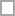# PRM Quizzes & Trivia

Professional Risk Manager’s (PRM) do exactly what the name suggests; they manage risk. Do you think you have what it takes to be a PRM? Are you looking for a career change or is this something you’ve always wanted to do? There is one fun way to find out if you’re ready. Take these quizzes full of helpful practice questions!

PRM’s have to pass a series of four exams to obtain their designation and validation of skills. What is the second exam in the series of four that you have to pass? What is a secondary risk? If management has set a recovery time objective (RTO) for an employee’s process, what does this mean?

What is a recovery point objective (RPO)? True or false: insurance is a way to transfer risk? What is the Delphi technique? Are you ready to take a risk and take these quizzes today?

A mental exercise about the "5 stans" and PRM.

Questions: 10  |  Attempts: 36   |  Last updated: Feb 14, 2013
• Sample Question
Gengghis Khan used this to justify his rulePRM Logistic Regression

Questions: 25  |  Attempts: 19   |  Last updated: Jan 28, 2013
• Sample Question
Logistic Regression (LR) is very similar to ___ except the predictors do not need to be ____.Statistical significance

Questions: 5  |  Attempts: 13   |  Last updated: Jan 16, 2013
• Sample Question
The statistical significance of a result is the :Related

• Gengghis Khan used this to justify his rulePRM question from

• Rulers of the Mongol EmpirePRM question from

• The Golden Horde is located inPRM question from

• Logistic Regression (LR) is very similar to ___ except the predictors do not need to be ____.PRM question from

• In LR, the DV is a ___ variable, even though it represents a ___ probabilistic occurence of an event occurring.PRM question from

• The 5 assumptions are (1) independence (2) linearity (3) normality (4) homogeneity of variance & (5) non-multicollinearity. LR does not require:PRM question from

• The statistical significance of a result is the :PRM question from

• In simpler terms, the stat signif of a result tells us something about :PRM question from

• The significance level (p) tells us :PRM question from